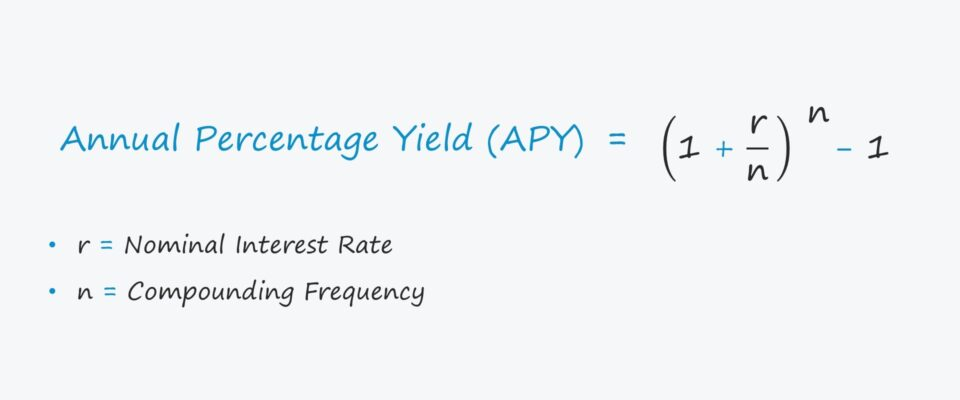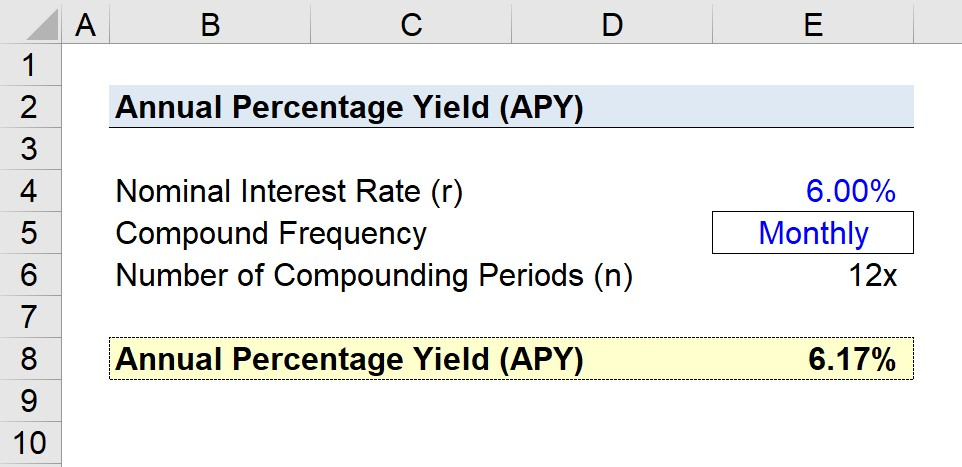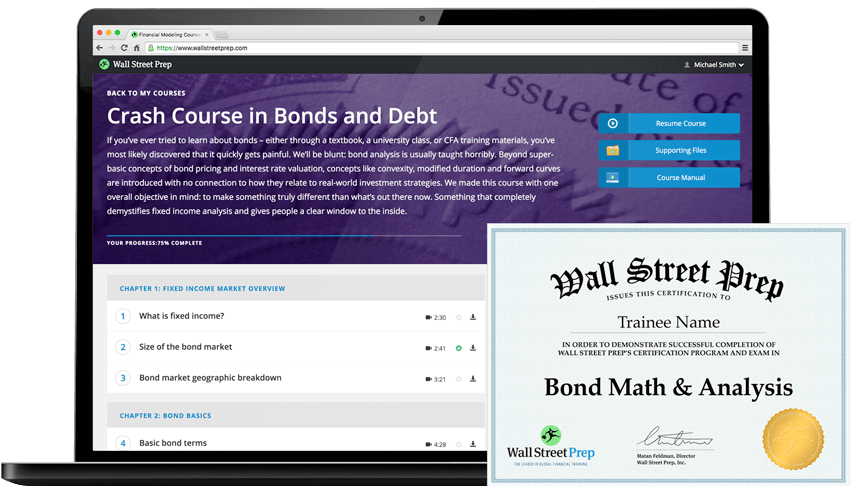# Annual Percentage Yield (APY)

Guide to Understanding the Annual Percentage Yield (APY)## How to Calculate APY (Step-by-Step)

APY stands for “Annual Percentage Yield” and measures the real amount of interest earned on an investment.

Often used interchangeably with the term “effective annual rate (EAR),” APY is the annualized return (or interest) earned from an investment.

In practice, APY is used to calculate the interest received from a deposit into a checking or savings account, certificate of deposit (CD), money market accounts, and other financial products.

Higher nominal interest rates and more compounding periods cause the APY (and returns) to increase – all else being equal.

The inclusion of the effects of compound interest is why the annual percentage yield (APY) is considered an accurate measure of returns.

By contrast, the principal amount remains the same when calculating simple interest – but for APY, the principal grows incrementally by the interest earned (i.e. “interest-on-interest”) – which is the concept of compound interest.

Therefore, APY specifically accounts for two major factors.

1. Nominal Interest Rate (%)
2. Frequency of Compounding

## APY Formula

The formula for calculating the annual percentage yield is as follows.

APY = [1 + (r ÷ n)] ^ n – 1

Where:

• r = Nominal Interest Rate
• n = Compounding Frequency

## Bank Deposit APY Calculation Example

For example, let’s say that you decided to deposit \$10,000 into a bank account with a nominal interest rate stated as 2.5%, which we’ll assume is compounded monthly.

Since there are twelve months in a year, the number of compounding periods (n) is twelve.

• Nominal Interest Rate (r) = 2.5%
• Compounding Frequency (n) = 12

The APY would be 2.53%, as shown by the equation below.

• APY = [1 + (2.5% ÷ 12)] ^ 12 – 1
• APY = 2.53%

## APR vs. APY: What is the Difference?

The annual percentage yield (APY) and annual percentage return (APR) are two frequently discussed terms, as both are annualized rates expressed as a percentage.

But APR is calculated to measure the interest to be paid (“owed”), whereas APY is used to estimate the interest to be received (“earned”).

The APY is the effective annual rate (EAR) that considers how frequently interest is applied to the principal balance.

On the other hand, the APR is the stated annual interest rate without the effects of compounding, i.e. the nominal interest rate.

Thus, the difference between APR and APY is that the latter reflects the effects of compound interest.

Unlike APY, the APR calculates the simple interest rate, so the variance between APR and APY widens if the interest rate is higher and the number of compounding periods is fewer.

For example, if an item was purchased for \$24,000 using a credit card with a 10% APR, the principal is \$24,000, and the buyer is charged \$2,400 in total interest for the next twelve months.

• Annual Interest = \$24,000 ×10% = \$2,400

If we divide the \$2,400 annual interest amount by twelve, we are left with \$200 as the interest owed per month.

• Monthly Interest = \$2,400 ÷ 12 = \$200

## APY Calculator – Excel Model Template

We’ll now move to a modeling exercise, which you can access by filling out the form below.Submitting ...

## APY Calculation Example

Suppose that you placed capital into an investment with a stated annual interest rate of 6% (i.e. nominal interest rate).

In order to observe the impact of compounding frequency and the variance between the APY and APR, we’ll create a toggle by clicking on “Data Validation” and creating a drop-down list with the following selections (i.e. the column on the left).

Compounding Frequency Number of Periods
Daily 365x
Monthly 12x
Quarterly 4x
Semi-Annually 2x
Annually 1x

In the cell to the right, we’ll use the “IF” function for the formula to output the corresponding number of compounding periods based on the active selection.

The annual percentage yield (APY) can now be calculated by entering our assumptions into the formula from earlier.

• Annual Percentage Yield (APY) = (1 + 6.00% ÷ n) ^ n – 1

At each of the different compounding frequency assumptions, we calculate the following APYs.

• Daily = 6.18%
• Monthly = 6.17%
• Quarterly = 6.14%
• Semi-Annually = 6.09%
• Annually = 6.00%

So from above, we can see how the increased frequency in compounding causes APY to rise from the interest being earned on the accrued interest to the principal.

The effect of compounding on APY could appear marginal, but across long time horizons and with a greater amount of capital invested, these minor differences can have significant implications on the long-term returns.Step-by-Step Online Course

### Crash Course in Bonds and Debt: 8+ Hours of Step-By-Step Video

A step-by-step course designed for those pursuing a career in fixed income research, investments, sales and trading or investment banking (debt capital markets).Inline Feedbacks';
Learn Financial Modeling Online

Everything you need to master financial and valuation modeling: 3-Statement Modeling, DCF, Comps, M&A and LBO.

X

The Wall Street Prep Quicklesson Series

7 Free Financial Modeling Lessons

Get instant access to video lessons taught by experienced investment bankers. Learn financial statement modeling, DCF, M&A, LBO, Comps and Excel shortcuts.